Get ICSE Solutions for Class 10 Mathematics Chapter 16 Constructions (Circle) for ICSE Board Examinations on ICSESolutions.com. We provide step by step Solutions for ICSE Mathematics Class 10 Solutions Pdf. You can download the Class 10 Maths ICSE Textbook Solutions with Free PDF download option.

## Constructions (Circle) Class 10 Maths ICSE Solutions

### Figure Based Questions

Question 1. Take a point O on the plane at the paper. With O as centre draw a circle of radius 3 cm. Take a point P on this circle and draw a tangent at P.
Solution: Steps of construction:
(i) Take a point O on the plane at the paper and draw a circle at radius 3 cm.Question 2. Four equal circles, each of radius 5 cm, touch each other as shown in the figure. Find the area included between them. (Take π= 3.14)Question 3. In the figure alongside, OAB is a quadrant of a circle. The radius OA = 3.5 cm and OD = 2 cm. Calculate the area of the shaded 22 portion.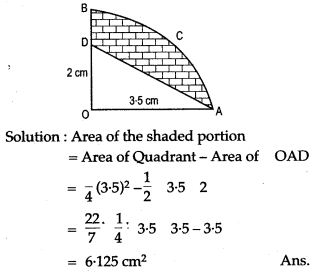Question 4. AC and BD are two perpendicular diameter of a circle ABCD. Given that the area of shaded portion is 308 cm2 calculate:Question 5. The diagram represents the area swept by wiper of a car. With the dimension given in figure, calculate the shaded swept by the wiper.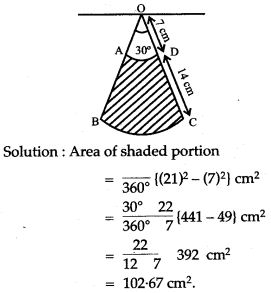Question 6. AC and BD are two perpendicular diameters of a circle with centre O. If AC = 16 cm, calculate the area and perimeter of the shaded part. (Take π = 3.14).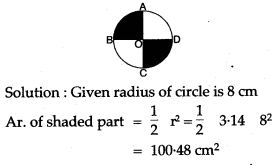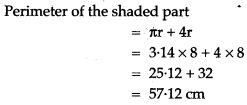Question 7. Draw a circle at radius 4 cm. Take a point on it. Without using the centre at the circle, draw a tangent to the circle at point P.Question 8. Draw a circle at radius 3 cm. Take a point at 5.5 cm from the centre at the circle. From point P, draw two tangent to the circle.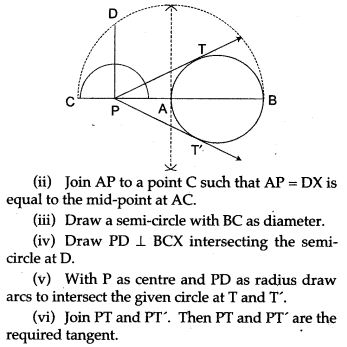Question 9. Use a ruler and a pair of compasses to construct ΔABC in which BC = 4.2 cm, ∠ ABC = 60° and AB 5 cm. Construct a circle of radius 2 cm to touch both the arms of ∠ ABC of Δ ABC.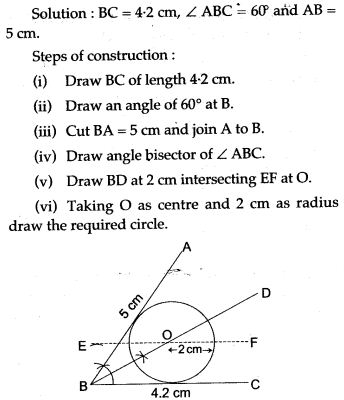Question 10. Construct an isosceles triangle ABC such that AB = 6 cm, BC = AC = 4 cm. Bisect ∠C internally and mark a point P on this bisector such that CP = 5 cm. Find the points Q and R which are 5 cm from P and also 5 cm from the line AB.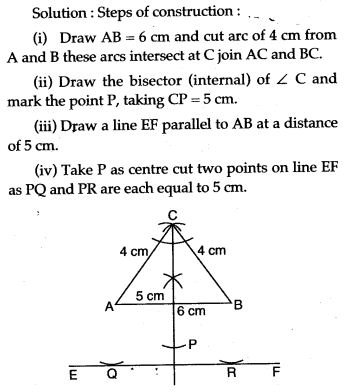Question 11. Draw two lines AB, AC so that ∠ B AC = 40°:
(i) Construct the locus of the centre of a circle that touches AB and has a radius of 3.5 cm.
(ii) Construct a circle of radius 35 cm, that touches both AB and AC, and whose centre lies within the ∠BAC.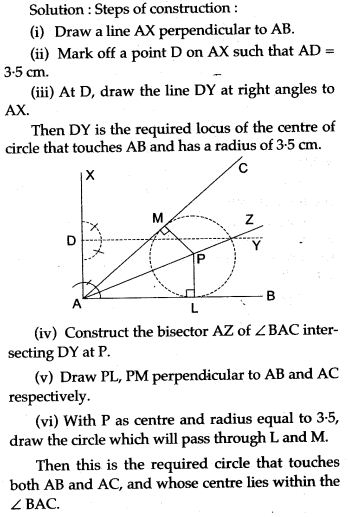Question 12. Draw a circle of radius 3.5 cm. Mark a point P outside the circle at a distance of 6 cm from the centre. Construct two tangents from P to the given circle. Measure and write down the length of one tangent.Question 13. Construct a triangle ABC, given that the radius of the circumcircle of triangle ABC is 3.5 cm, ∠ BCA = 45° and ∠ BAC = 60°.
Solution: Steps of construction: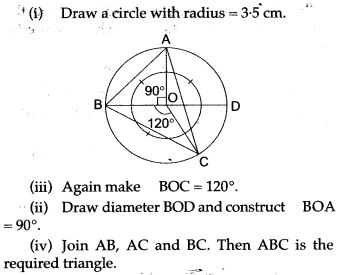Question 14. Construct an angle PQR = 45°. Mark a point S on QR such that QS = 4.5 cm. Construct a circle to touch PQ at Q and also to pass through S.Question 15. Construct the circumcircle of the ABC when BC = 6 cm, B = 55° and C = 70°.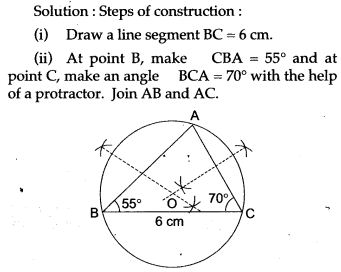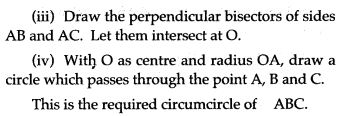Question 16. Using ruler and compass only, construct a triangle ABC such that AB = 5 cm, ABC = 75° and the radius of the circumcircle of triangle ABC is 3.5 cm.
On the same diagram, construct a circle, touching AB at its middle point and also touching the side AC.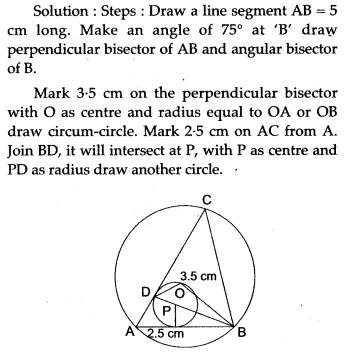Question 17. (a) Only ruler and compass may be used in this question. All contraction lines and arcs must be clearly shown and be of sufficient length and clarity to permit assessment.
(i) Construct a ABC, such that AB = AC = 7 cm and BC = 5 cm.
(ii) Construct AD, the perpendicular bisector of BC.
(iii) Draw a circle with centre A and radius 3 cm. Let this drcle cut AD at P.
(iv) Construct another circle, to touch the circle with centre A, externally at P, and pass through B and C.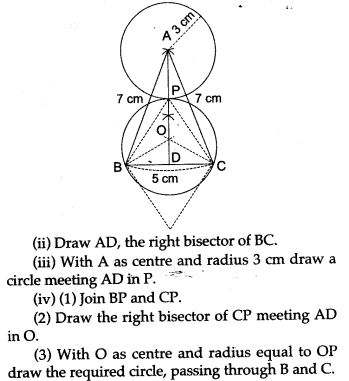Question 18. Using ruler and compass construct a cyclic quadrilateral ABCD in which AC = 4 cm, ∠ ABC = 60°, AB 1.5 cm and AD = 2 cm. Also write the steps of construction.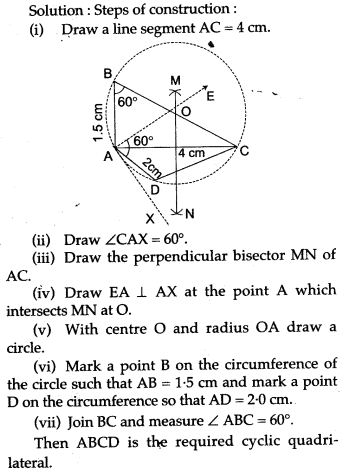Question 19. Construct a triangle whose sides are 4.4 cm, 5.2 cm and 7.1 cm. Construct its circumcircle. Write also the steps of construction.
Solution: Steps of construction:Question 20. Draw a circle of radius 3 cm. Construct a square about the circle.Question 21. Draw a circle of radius 2.5 cm and circumscribe a regular hexagon about it.Question 22. Construct the rhombus ABCD whose diagonals AC and BD are of lengths 8 cm and 6 cm respectively. Construct the inscribed circle of the rhombus. Measure its radius.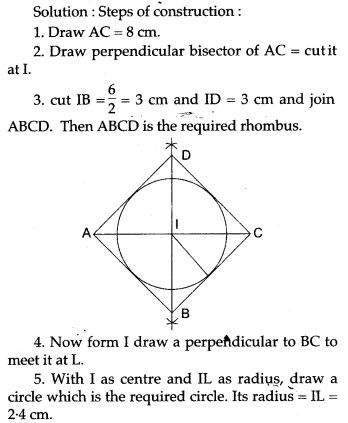Question 23. Draw an isosceles triangle with sides 6 cm, 4 cm and 6 cm. Construct the in circle of the triangle. Also write the steps of construction.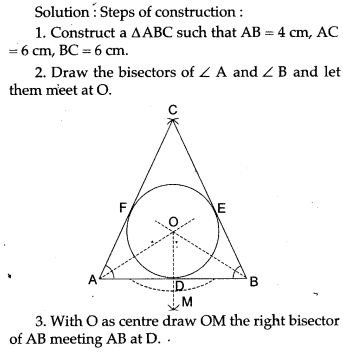Question 24. Use ruler and compasses only for this question:
(i) Construct A ABC, where AB = 3.5 cm, BC = 6 cm and ∠ ABC = 60°.
(ii) Construct the locus of points inside the triangle which are equidistant from BA and BC.
(iii) Construct the locus of points inside the triangle which are equidistant from B and C.
(iv) Mark the point P which is equidistant from AB, BC and also equidistant from B and C. Measure and record the length of PB.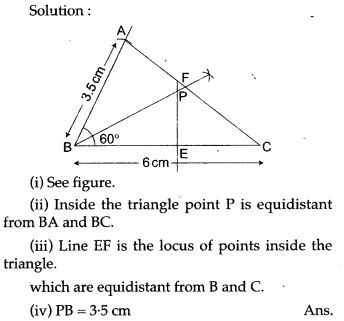Question 25. Construct a Δ ABC with BC = 6.5 cm, AB = 5.5 cm, AC = 5 cm. Construct the incircle of the triangle. Measure and record the radius of the incircle.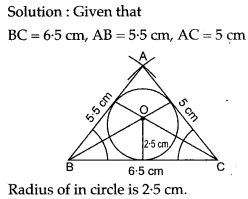Question 26. Draw a circle of radius 4 cm. Take a point P out side the circle without using the centre at the circle. Draw two tangent to the circle from point P.
Solution: Steps of construction:
(i) Draw a circle of radius 4 cm.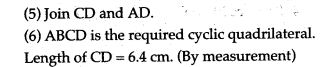Question 28. Ruler and compasses only may be used in this question. All constructions lines and arcs must be clearly shown, and the be sufficient length and clarity to permit assessment:
(i) Construct a triangle ABC, in which AB = 9 cm, BC = 10 cm and angle ABC = 45°.
(ii) Draw a circle, with centre A and radius 2.5 cm. Let it meet AB at D.
(iii) Construct a circle to touch the circle with center A externally at D and also to touch the line BC.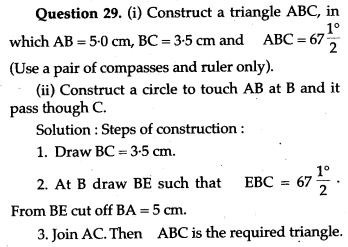Question 30. The centre O of a circle of a radius 1.3 cm is at a distance of 3.8 cm from a given straight line AB. Draw a circle to touch the given straight line AB at a point P so that OP = 4.7 cm and to touch the given circle externally.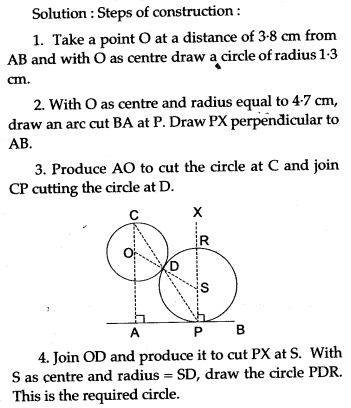Question 31. Construct a triangle having base 6 cm, vertical angle 60° and median through the vertex is 4 cm.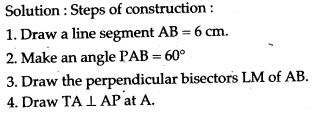Question 32. Using a ruler and compasses only:
(i) Construct a triangle ABC with the following data:
AB = 3.5 cm, BC = 6 cm and ∠ ABC = 120°.
(ii) In the same diagram, draw a circle with BC as diameter. Find a point P on the circumference of the circle which is equidistant from AB and BC.
(iii) Measure ∠ BCP.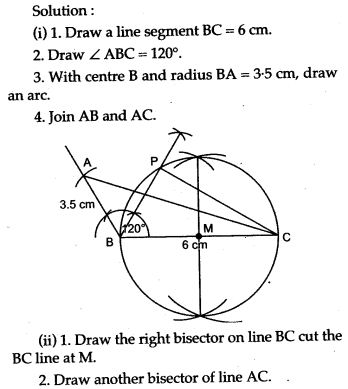Question 33. Draw a circle of radius 3 cm and construct a tangent to it from an external point without using the centre.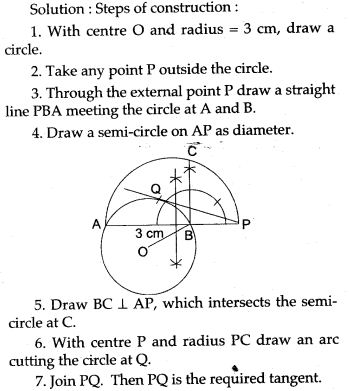Question 34. Construct a ΔABC with base BC = 3.5 cm, vertical angle ∠BAC = 45° and median through the vertex A is 3.5 cm. Write also the steps of construction.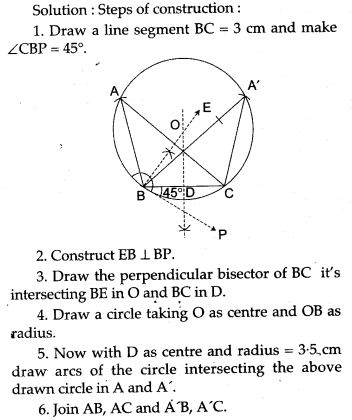For More Resources